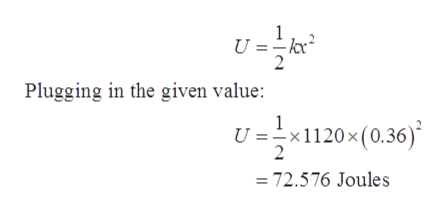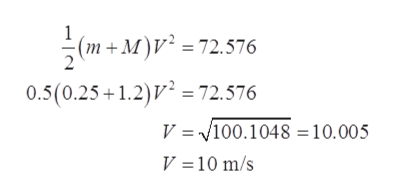# A 0.250 kg dart is fired into a stationary 1.20 kg block of wood that is on a frictionless surface (of course!). The dart and block then impact a spring with a spring constant of k = 1120 N/m and compresses it 0.360 m. (Use g = 10 m/s2, as needed. Do not enter units in numerical answers.)What is the total energy stored in the spring, in Joules, when it is fully compressed? ____ What is the speed, in m/s, of the dart-block combination after the collision? ____ How fast was the dart moving, in m/s, before it hit the block of wood?

Question
2 views

A 0.250 kg dart is fired into a stationary 1.20 kg block of wood that is on a frictionless surface (of course!). The dart and block then impact a spring with a spring constant of k = 1120 N/m and compresses it 0.360 m. (Use g = 10 m/s2, as needed. Do not enter units in numerical answers.)

What is the total energy stored in the spring, in Joules, when it is fully compressed? ____

What is the speed, in m/s, of the dart-block combination after the collision? ____

How fast was the dart moving, in m/s, before it hit the block of wood?

check_circle

Step 1

Given values:

Mass of dart, m = 0.25 kg

Mass of block, M = 1.20 kg

Spring constant, k = 1120 N/m

Compression in the spring, x = 0.360 m

Step 2

(a)

Energy stored in the spring can be calculated by the formula:help_outlineImage TranscriptioncloseU =: kx Plugging in the given value: 1 U =x1120x (0.36) 2 72.576 Joules fullscreen
Step 3

(b)

Let the speed of the dart - block system be V

From conservation of energy:

&n...help_outlineImage Transcriptionclose(m + M)V2 = 72.576 0.5(0.25+1.2)272.576 V = 100.1048 10.005 V = 10 m/s fullscreen

### Want to see the full answer?

See Solution

#### Want to see this answer and more?

Solutions are written by subject experts who are available 24/7. Questions are typically answered within 1 hour.*

See Solution
*Response times may vary by subject and question.
Tagged in

### Physics